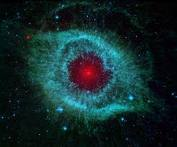Sunday 13 October 2019

IRON’S ATOMIC NUMBER AND ISOTOPESThis post is also available in: German, Albanian, Kurdish, Turkish

Let us see first the verse that mentions the importance and characteristics of iron:

25- We have surely sent Our messengers with clear signs, and sent with them the book and the balance, so that man may stand by justice; and We sent down the iron, wherein there is strength, and many benefits for the people. So that God would know who will support Him and His messengers in secret. Verily God is Powerful, Almighty.
57-Iron, 25

The verb “inzal” in the Quran is usually used to describe the action of coming from above the earth. The temperature of the earth was not sufficient, at the beginning, for the formation of iron. Not only the earth, but even a middle-sized star like our sun did not have the heat necessary for the formation of iron. That is why iron must have come to our planet, as well as to the entire solar system, from outer space. The iron we have in our planet today must have come to our solar system from other stars that had higher temperatures favorable for the formation of iron. Can it be possible that while describing the formation of the iron, the Quran’s drawing attention to iron’s landing from outside to our world by the word “inzal” is to emphasize this phenomenon?

The Quran shows other mathematical miracles by pointing out the iron’s atomic number, which is 26, in different ways.

1. In the Quran, there is a mathematical value for every letter. (We gave all the letters and their numerical values in the chapters on 19). The mathematical value for the word iron (hadid) is 26.

 Ha = 8 Da = 4 Ya = 10 Da = 4 Total = 26

2. The verse that refers to iron is the 25th verse in the sura “Hadid”. If we count Basmalah, then that number is 26.

3. The name “God” mentioned in this sura for the 26th time is in the same verse. The atomic number of an element is the main characteristic of that element and is determined by the number of its protons, which are the building blocks of that element. Iron’s atomic number is 26 because of its 26 protons. From the beginning of the sura “Iron” (Hadid) till the end of the verse of that sura that alludes to iron, the word “God” is used 26 times.

 The word Mathematical value of the word Iron 26

 Iron’s atomic number 26 How many times is the word “God” used from the beginning of the sura until the end of the 25th verse, which is the only verse wherein the properties of iron are mentioned? 26

As iron’s atomic number is encoded in the sura that mentions iron, there is also a sign in that sura about iron’s isotopes.

The word “al hadid,” which refers to a particular iron, has a mathematical value of 57. The article “al” corresponds to “the” in English. When the word “hadid” is used with the article “al,” referring to a specific iron, the mathematical value turns out to be 57.

 Alif = 1 Lam = 30 Ha = 8 Dal = 4 Ya = 10 Dal = 4 Total = 57

1. The sura “Iron” (Hadid) is the 57th sura of the Quran. And 57 is one of the isotopes of iron.

2. The sura “Iron” (Hadid) is the 58th sura from the end of the Quran. That is another isotope of iron.

3. This sura has 29 numbered verses. This number becomes 30 when the unnumbered Basmalah is counted. These two numbers (29 and 30) are equal to the neutron numbers of two isotopes of iron, from the total of four. The frequency of the word “God” in this sura gives the neutron number of the other isotope.

 The word Mathematical value of the word The iron (al hadid) 57

 One of iron’s isotopes 57 What is the sura number of the sura the Iron? 57